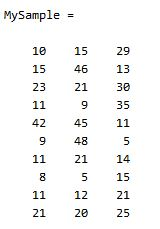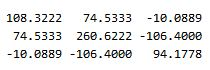CODING MY LIFE

0%

### 统计学的基本概念

• 均值：$\bar{X}=\frac{\sum\_{i=1}^n X\_{i}}{n}$
• 标准差：$s=\sqrt{\frac{\sum\_{i=1}^n (X\_{i}-\bar{X})^2}{n-1}}$
• 方差：$s^2=\frac{\sum\_{i=1}^n (X\_{i}-\bar{X})^2}{n-1}$

### 协方差多了就是协方差矩阵### Matlab 协方差实战Update：今天突然发现，原来协方差矩阵还可以这样计算，先让样本矩阵中心化，即每一维度减去该维度的均值，使每一维度上的均值为 0，然后直接用新的到的样本矩阵乘上它的转置，然后除以(N-1)即可。其实这种方法也是由前面的公式通道而来，只不过理解起来不是很直观，但在抽象的公式推导时还是很常用的！同样给出 Matlab 代码实现：

### 总结

P.S.写论文要选 Latex，在 wordpress 里编辑公式还得用Latex，用 Latex 还真对得起咱学计算机这张脸~